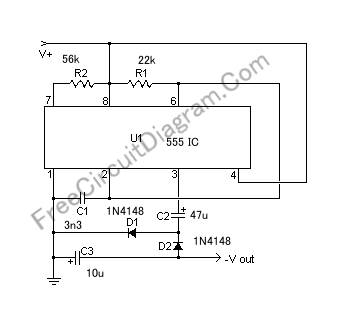# Simple 555 Switching Circuit Provides Negative Supply from Single Positive SupplyIf you need a negative supply for op-amp or just need negative bias voltage while operating from a single supply voltage (for battery operation for example), this circuit helps providing the negative supply for you. The circuit is based on charge pump driven by 555 switching circuit. Here is the schematic diagram:The negative voltage produced by this circuit is about 1 volt smaller than the positive supply voltage magnitude. If you use 9V positive supply then the negative output will be around 8 volt, or if you use 12 volt positive supply the you’ll get around 11Volt negative supply. 100mA current could be drawn safely from the negative voltage output. This is enough for most op-amp or other small signal application.